# Chemical Equations Reactions Chemistry Chapter 8 Chemical Reactions

• Slides: 51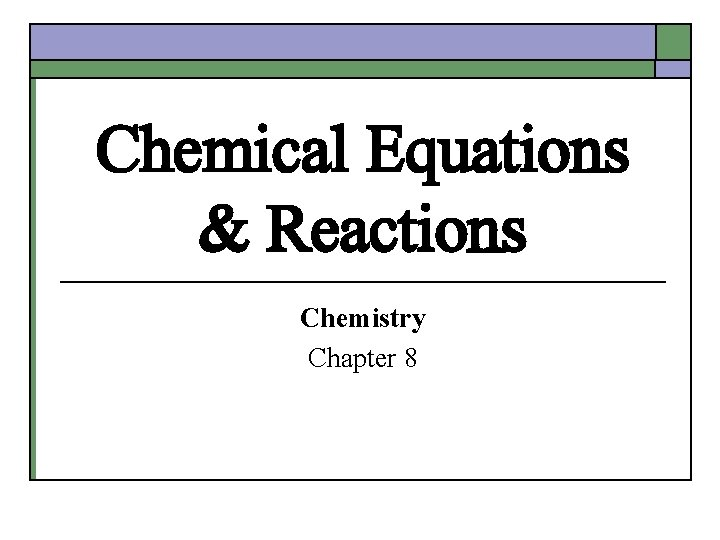Chemical Equations & Reactions Chemistry Chapter 8Chemical Reactions A. B. Definition: a process by which 1 or more substances, called reactants, are changed into 1 or more substances, called products, with different physical & chemical properties. Evidence of a Chemical Reaction 1. 2. 3. 4. 5. Color change Formation of a precipitate, ppt Release of a gas Energy change – heat, light, sound Odor change Reactions are started by the addition of energy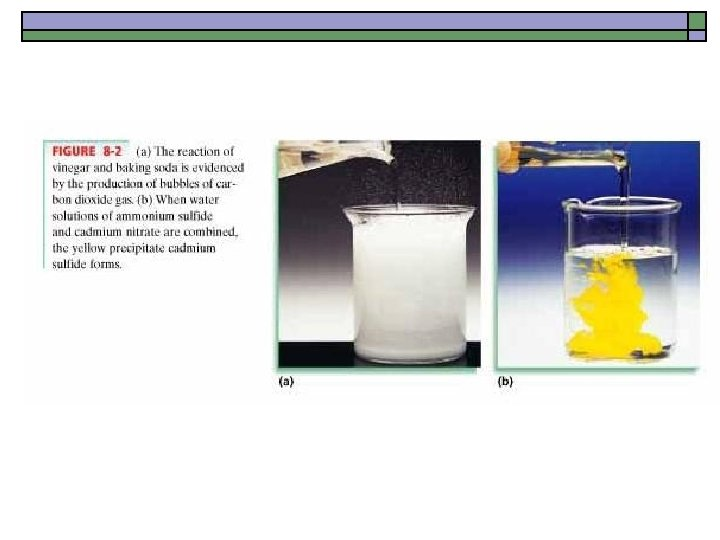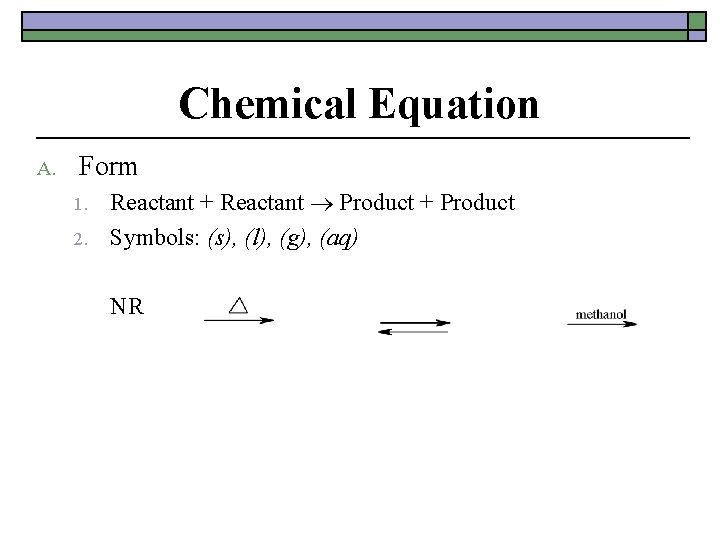Chemical Equation A. Form 1. 2. Reactant + Reactant Product + Product Symbols: (s), (l), (g), (aq) NR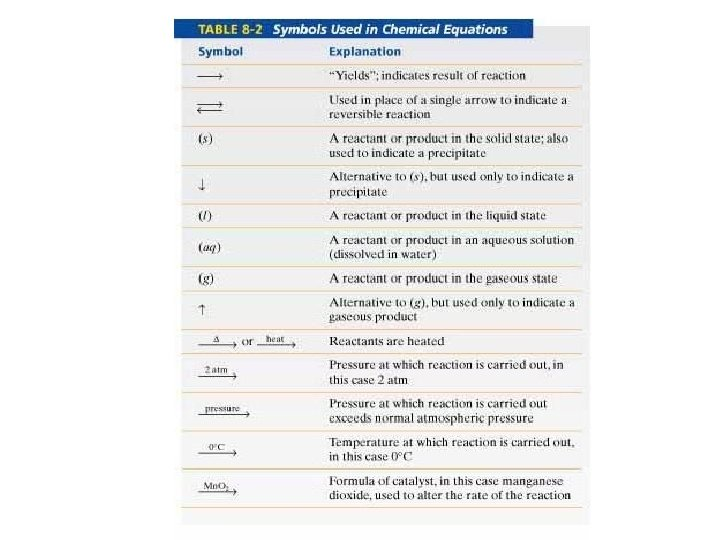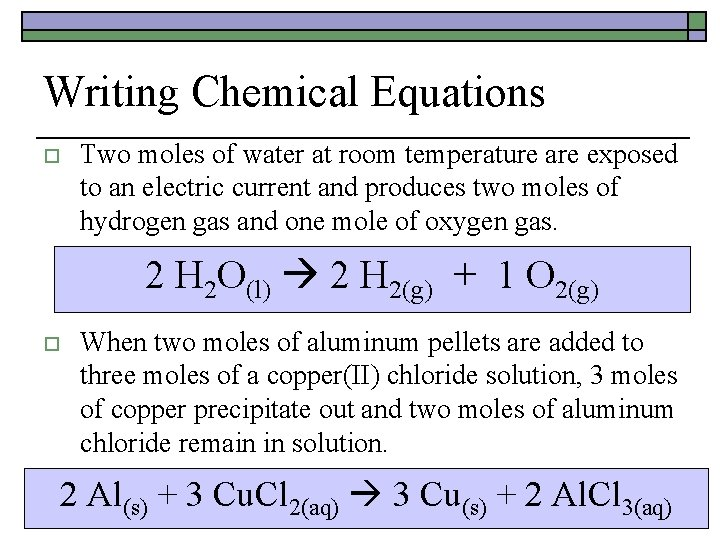Writing Chemical Equations o Two moles of water at room temperature are exposed to an electric current and produces two moles of hydrogen gas and one mole of oxygen gas. 2 H 2 O(l) 2 H 2(g) + 1 O 2(g) o When two moles of aluminum pellets are added to three moles of a copper(II) chloride solution, 3 moles of copper precipitate out and two moles of aluminum chloride remain in solution. 2 Al(s) + 3 Cu. Cl 2(aq) 3 Cu(s) + 2 Al. Cl 3(aq)Characteristics of A Balanced Chemical Equations 1. 2. 3. 4. The equation must represent known facts. All substances have been identified. The equation must contain the correct symbols and/or formulas for the reactants and products Can be either a word equation or a formula equation The law of conservation of mass must be satisfied. This provides the basis for balancing chemical equations. 1 st formulated by Antoine Lavoisier TOTAL MASS REACTANTS = TOTAL MASS PRODUCTS Number of atoms of EACH element is the SAME on both sides of the equation.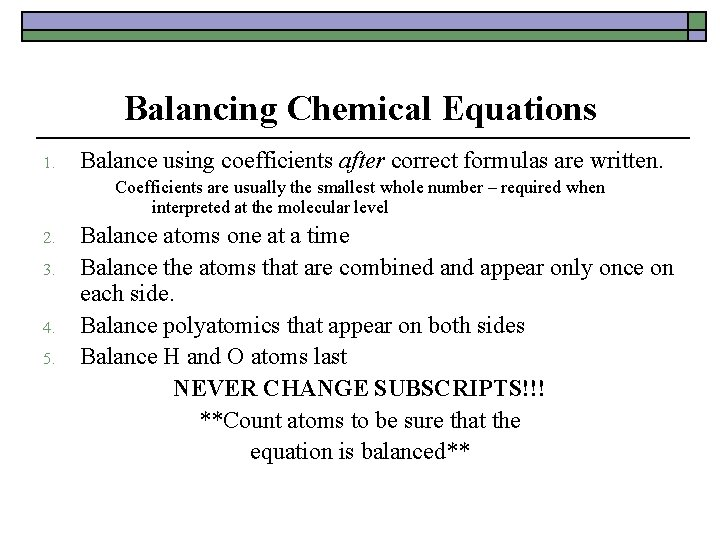Balancing Chemical Equations 1. Balance using coefficients after correct formulas are written. Coefficients are usually the smallest whole number – required when interpreted at the molecular level 2. 3. 4. 5. Balance atoms one at a time Balance the atoms that are combined and appear only once on each side. Balance polyatomics that appear on both sides Balance H and O atoms last NEVER CHANGE SUBSCRIPTS!!! **Count atoms to be sure that the equation is balanced**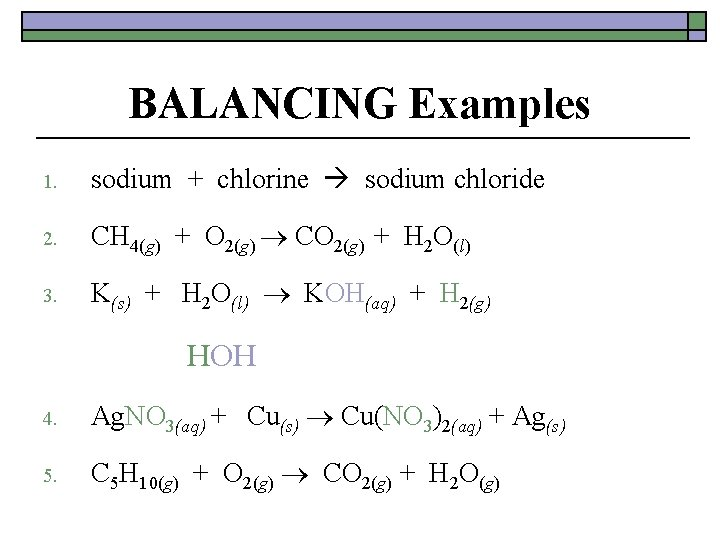BALANCING Examples 1. sodium + chlorine sodium chloride 2. CH 4(g) + O 2(g) CO 2(g) + H 2 O(l) 3. K(s) + H 2 O(l) KOH(aq) + H 2(g) HOH 4. Ag. NO 3(aq) + Cu(s) Cu(NO 3)2(aq) + Ag(s) 5. C 5 H 10(g) + O 2(g) CO 2(g) + H 2 O(g)Interpretation of a Balanced Equation 2 Mg(s) + O 2(g) 2 Mg. O(s) 2 atoms of solid magnesium react with 1 molecule of oxygen gas to form 2 formula units of solid magnesium oxide OR 2 moles of solid magnesium react with 1 moles of oxygen gas to form 2 moles of solid magnesium oxide Reaction Ratios:Classifying Chemical Reactions A. Pattern for prediction based on the kind of reactants Combustion or Burning – complete combustion always produces carbon dioxide and water! 1. Hydrocarbons Cx. Hy + O 2 CO 2 + H 2 O 2. Alcohols Cx. Hy. OH + O 2 CO 2 + H 2 O 3. Sugars C 6 H 12 O 6 + O 2 CO 2 + H 2 O C 12 H 22 O 11 + O 2 CO 2 + H 2 O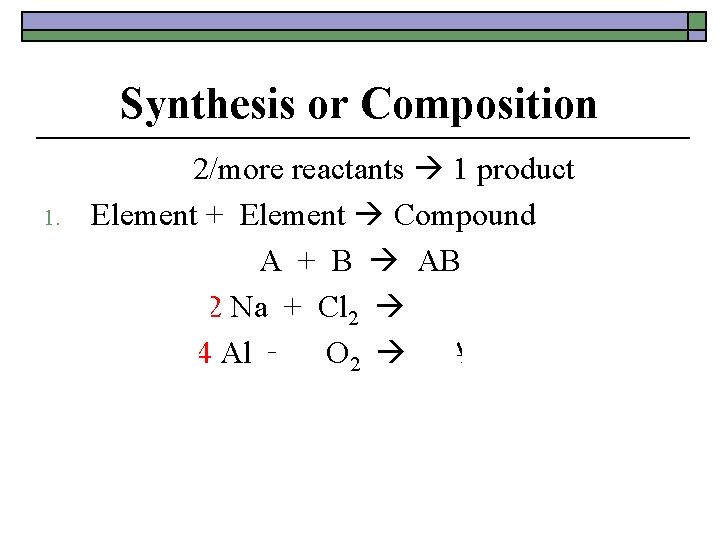Synthesis or Composition 1. 2/more reactants 1 product Element + Element Compound A + B AB 2 Na + Cl 2 2 Na. Cl 4 Al + 3 O 2 2 Al 2 O 3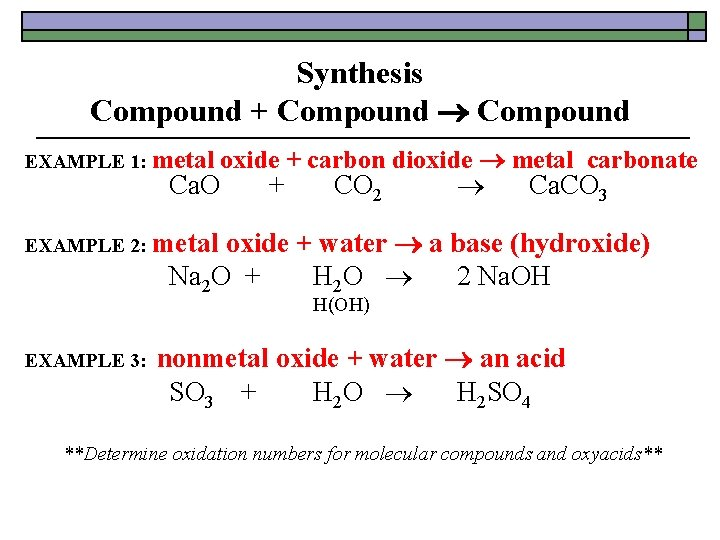Synthesis Compound + Compound EXAMPLE 1: metal oxide + carbon dioxide metal carbonate Ca. O + CO 2 Ca. CO 3 oxide + water a base (hydroxide) Na 2 O + H 2 O 2 Na. OH EXAMPLE 2: metal H(OH) EXAMPLE 3: nonmetal oxide + water an acid SO 3 + H 2 O H 2 SO 4 **Determine oxidation numbers for molecular compounds and oxyacids**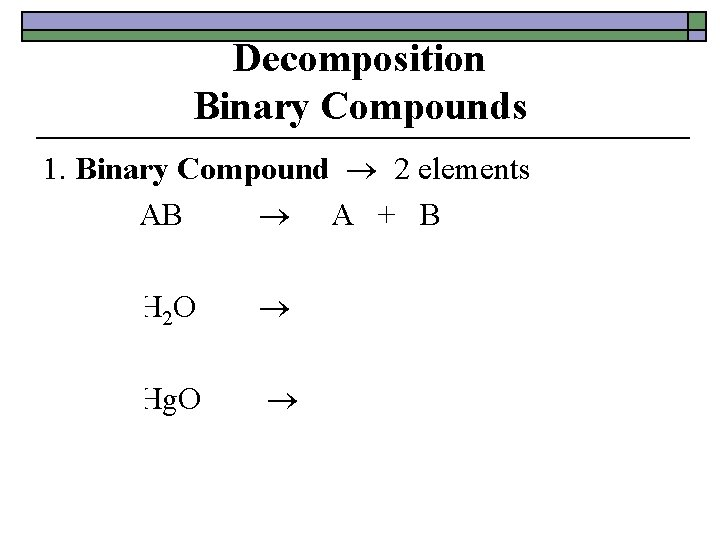Decomposition Binary Compounds 1. Binary Compound 2 elements AB A + B 2 H 2 O 2 Hg + O 2 2 H 2 + O 2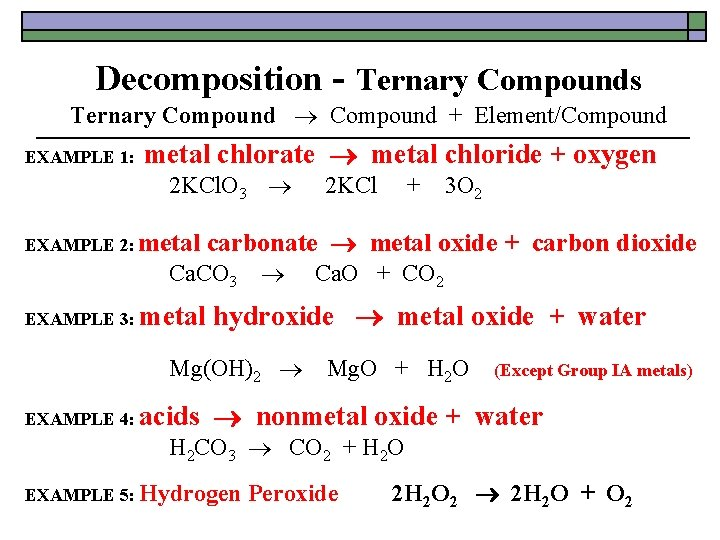Decomposition - Ternary Compounds Ternary Compound + Element/Compound EXAMPLE 1: metal chlorate metal chloride + oxygen 2 KCl. O 3 2 KCl + 3 O 2 EXAMPLE 2: metal Ca. CO 3 Ca. O + CO 2 EXAMPLE 3: metal hydroxide metal oxide + water Mg(OH)2 Mg. O + H 2 O (Except Group IA metals) EXAMPLE 4: acids carbonate metal oxide + carbon dioxide nonmetal oxide + water H 2 CO 3 CO 2 + H 2 O EXAMPLE 5: Hydrogen Peroxide 2 H 2 O 2 2 H 2 O + O 2Single Replacement or Single Displacement Element + Compound New Compound + New Element Metals 1. A + BC AC + B Active metals displace less active metals or hydrogen from their compounds in aqueous solution. Refer to the Activity Series. a. 2 Al + 3 Cu. Cl 2 2 Al. Cl 3 + 3 Cu b. metal + H 2 O metal hydroxide + H 2 An active metal (top of series to calcium) will react with water to form the hydroxide of the metal and hydrogen gas. 2 Na + 2 HOH 2 Na. OH + H 2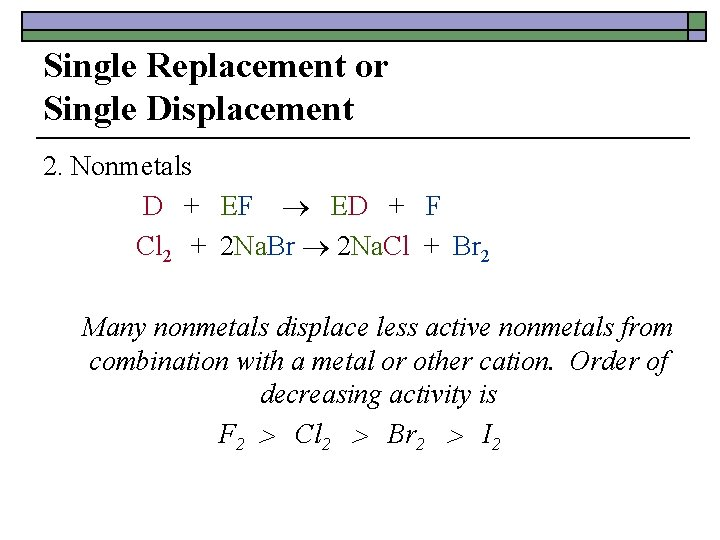Single Replacement or Single Displacement 2. Nonmetals D + EF ED + F Cl 2 + 2 Na. Br 2 Na. Cl + Br 2 Many nonmetals displace less active nonmetals from combination with a metal or other cation. Order of decreasing activity is F 2 Cl 2 Br 2 I 2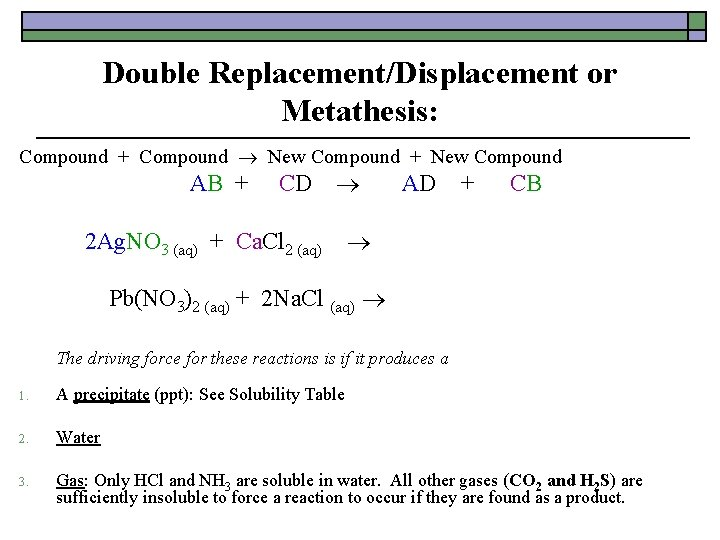Double Replacement/Displacement or Metathesis: Compound + Compound New Compound + New Compound AB + CD AD + CB 2 Ag. NO 3 (aq) + Ca. Cl 2 (aq) 2 Ag. Cl (s) + Ca(NO 3)2 (aq) Pb(NO 3)2 (aq) + 2 Na. Cl (aq) Pb. Cl 2 (s) + 2 Na. NO 3 (aq) The driving force for these reactions is if it produces a 1. A precipitate (ppt): See Solubility Table 2. Water 3. Gas: Only HCl and NH 3 are soluble in water. All other gases (CO 2 and H 2 S) are sufficiently insoluble to force a reaction to occur if they are found as a product.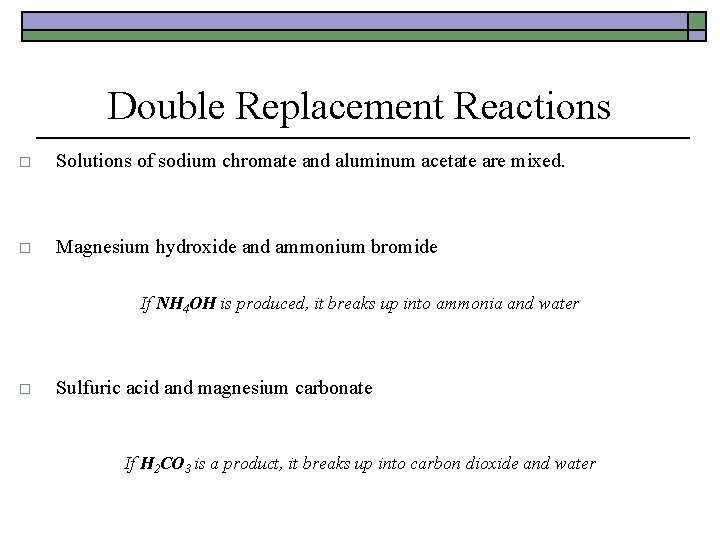Double Replacement Reactions o Solutions of sodium chromate and aluminum acetate are mixed. 3 Na 2 Cr. O 4(aq) + 2 Al(CH 3 COO)3(aq) + Al 2(Cr. O 4)3(s) + 6 Na. CH 3 COO(aq) o Magnesium hydroxide and ammonium bromide Mg(OH)2 + 2 NH 4 Br Mg. Br 2 + 2 NH 4 OH If NH 4 OH is produced, it breaks up into ammonia and water Mg(OH)2 + 2 NH 4 Br Mg. Br 2 + 2 NH 3 + 2 H 2 O o Sulfuric acid and magnesium carbonate H 2 SO 4 + Mg. CO 3 Mg. SO 4 + H 2 CO 3 If H 2 CO 3 is a product, it breaks up into carbon dioxide and water H 2 SO 4 + Mg. CO 3 Mg. SO 4 + H 2 O + CO 2Thermochemistry o o The study of the changes in energy that accompany a chemical reaction and physical changes. Chemical Reactions involve changes in energy that result from o o o Bond breaking that requires energy (absorbs) from the surroundings. Bond making that produces energy (releases) to the surroundings. Changes in energy result in an energy flow or transfer.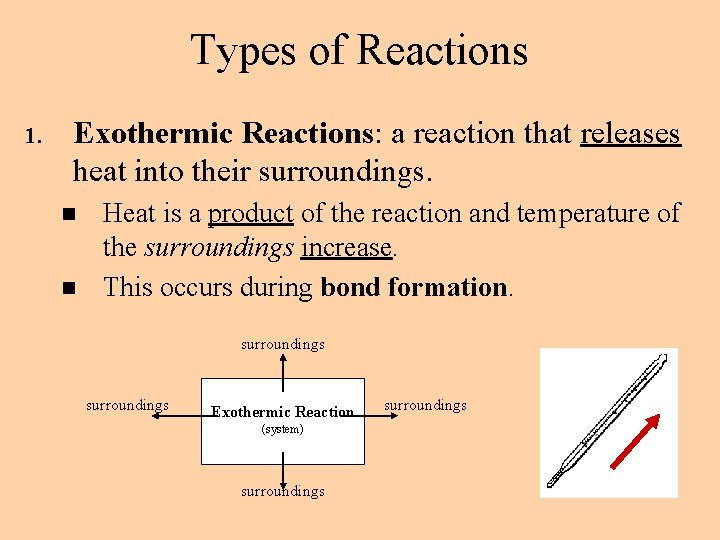Types of Reactions 1. Exothermic Reactions: a reaction that releases heat into their surroundings. n n Heat is a product of the reaction and temperature of the surroundings increase. This occurs during bond formation. surroundings Exothermic Reaction (system) surroundingsTypes of Reactions 2. Endothermic Reactions: a reaction that absorbs heat from the surroundings. n n Heat acts as a reactant and temperature of the surroundings decreases. This occurs during bond breaking. surroundings Endothermic Reaction (system) surroundings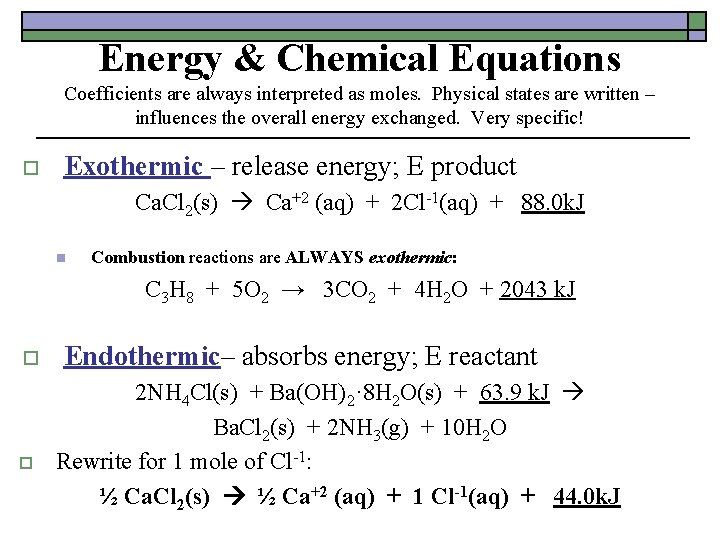Energy & Chemical Equations Coefficients are always interpreted as moles. Physical states are written – influences the overall energy exchanged. Very specific! o Exothermic – release energy; E product Ca. Cl 2(s) Ca+2 (aq) + 2 Cl-1(aq) + 88. 0 k. J n Combustion reactions are ALWAYS exothermic: C 3 H 8 + 5 O 2 → 3 CO 2 + 4 H 2 O + 2043 k. J o o Endothermic– absorbs energy; E reactant 2 NH 4 Cl(s) + Ba(OH)2· 8 H 2 O(s) + 63. 9 k. J Ba. Cl 2(s) + 2 NH 3(g) + 10 H 2 O Rewrite for 1 mole of Cl-1: ½ Ca. Cl 2(s) ½ Ca+2 (aq) + 1 Cl-1(aq) + 44. 0 k. JHeat and Enthalpy Changes o Enthalpy (H): the heat content of a system at constant pressure. n o Unit: J Enthalpy Change ( H): is the heat absorbed or released in a physical or chemical change at constant pressure. n n H = Hproducts ─ Hreactants This can be measured. H is also known as the heat of the reaction. Difference between the stored energy of the reactants and the products.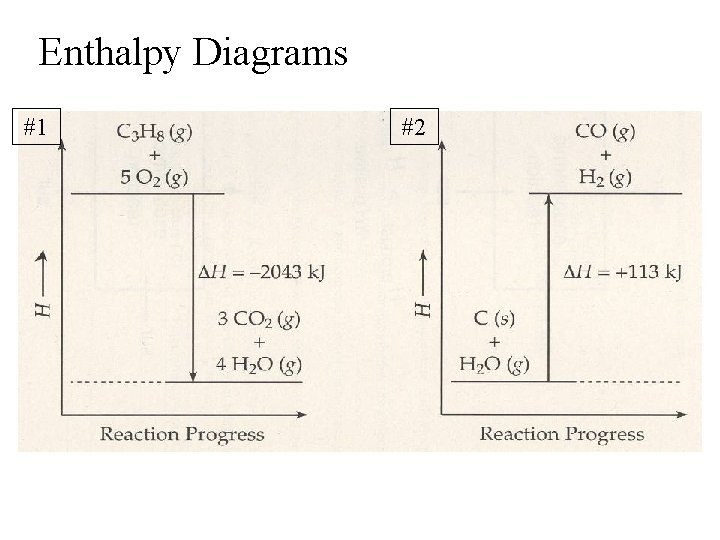Enthalpy Diagrams #1 #2#1 #2 a. Which has a higher enthalpy? Products or Reactants R P b. Was heat absorbed or released? R A c. Is this an endothermic or exothermic reaction? Exo Endo d. Is ΔH for this reaction positive or negative? - + e. Would the ΔH be on the left or right side of the yield sign? R L f. Is the reverse reaction exothermic or endothermic? Endo ExoRewrite each equation with the heat term in the reaction as a reactant or product – THERMOCHEMICAL equation: #1) C 3 H 8 + 5 O 2 → 3 CO 2 + 4 H 2 O + 2043 k. J #2) C + H 2 O + 113 k. J → CO + H 2Enthalpy Diagrams products reactants Ba. Cl 2 + 2 NH 3 + 10 H 2 O H (k. J) Ca. Cl 2 H (k. J) ∆H = --88. 0 k. J ∆H = ++63. 9 k. J reactants 2 NH 4 Cl + Ba(OH)2 8 H 2 O products Ca+2 + 2 Cl- Course of Reaction Endothermic Exothermic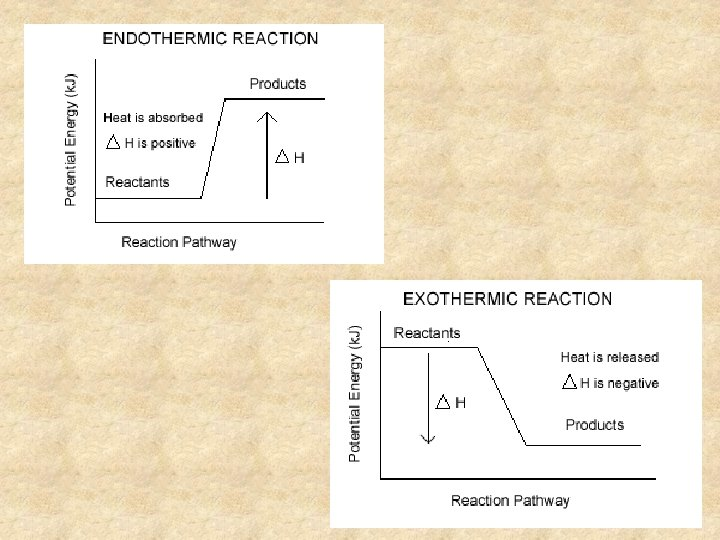Reaction Progress Collision Theory A. In order for a reaction to occur, the particles must collide A successful or effective collision occurs when 1. 2. a) b) 3. 4. The collision is energetic enough The particles collide with the correct orientation During a collision, kinetic energy is converted to potential energy The minimum energy needed for a successful collision = activation energy (Ea)Reaction Pathways or Potential Energy (heat content) DiagramsReaction Pathways or Potential Energy (heat content) Diagrams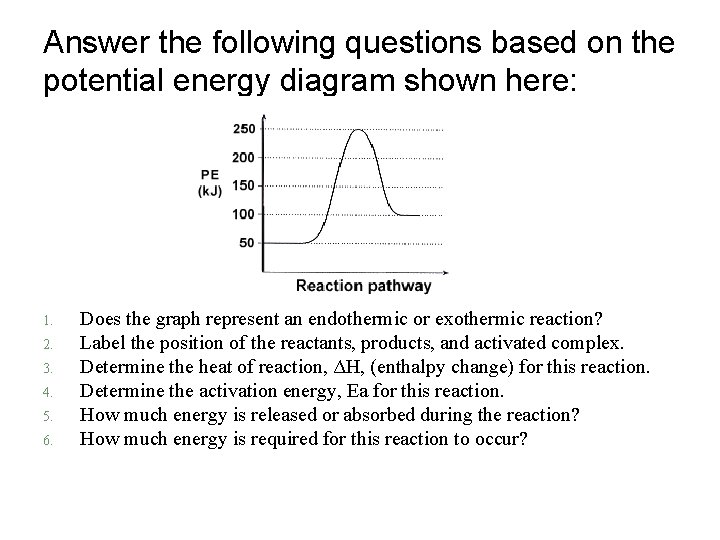Answer the following questions based on the potential energy diagram shown here: 1. 2. 3. 4. 5. 6. Does the graph represent an endothermic or exothermic reaction? Label the position of the reactants, products, and activated complex. Determine the heat of reaction, ΔH, (enthalpy change) for this reaction. Determine the activation energy, Ea for this reaction. How much energy is released or absorbed during the reaction? How much energy is required for this reaction to occur?Solution 1. The graph represents an endothermic reaction 2. 3. 4. 5. 6. ΔH = +50 k. J. Ea = +200 k. J 50 k. J of energy are absorbed during this endothermic reaction (this is the value of ΔH) 200 k. J of energy are required for this reaction to occur (Ea).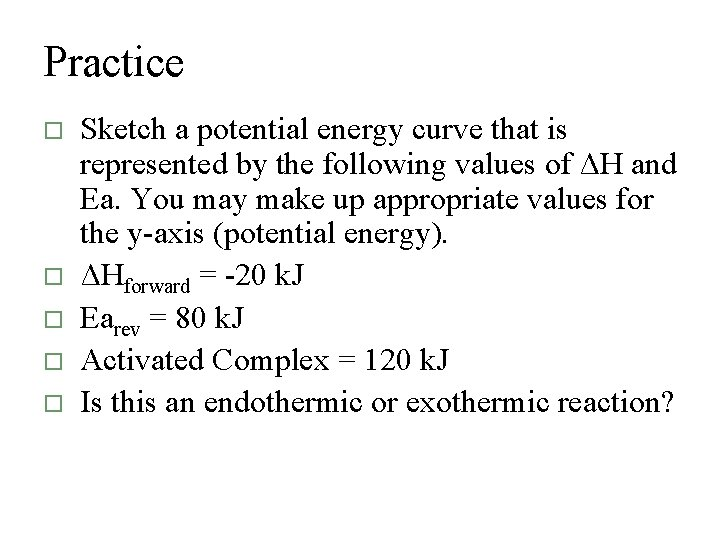Practice o o o Sketch a potential energy curve that is represented by the following values of ΔH and Ea. You may make up appropriate values for the y-axis (potential energy). ΔHforward = -20 k. J Earev = 80 k. J Activated Complex = 120 k. J Is this an endothermic or exothermic reaction?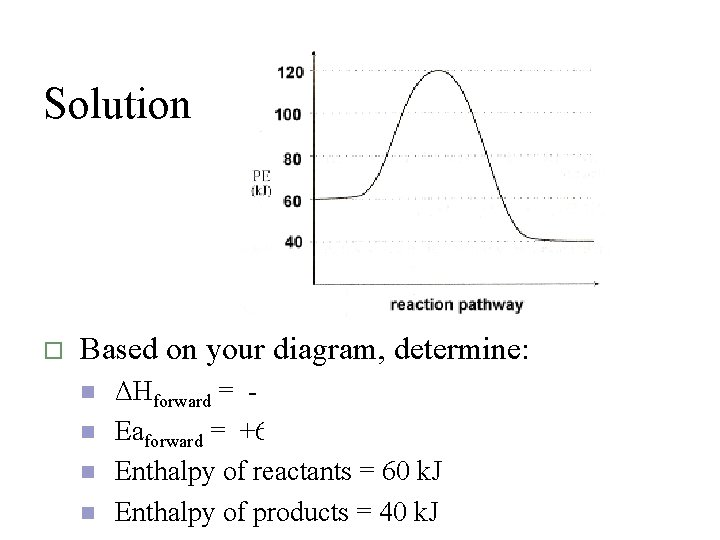Solution o Based on your diagram, determine: n n ΔHforward = -20 k. J Eaforward = +60 k. J Enthalpy of reactants = 60 k. J Enthalpy of products = 40 k. JEnthalpy Diagram - Formative Assessment #1 o o o Sketch a potential energy curve that is represented by the following values of ΔH and Ea. ΔHreverse = -10 k. J Eaforward = +40 k. J Activated Complex = 50 k. J Is this an endothermic or exothermic reaction?Enthalpy Diagram - Formative Assessment #1 Based on your diagram, determine: 1. 2. 3. 4. 5. Endo or Exo? ΔHforward = Eaforward = ΔHreverse = Eareverse =Enthalpy Diagram – FA #1 Answer Based on your diagram, determine: 1. 2. 3. 4. 5. Exothermic ΔHforward = -20 k. J Eaforward = +60 k. J ΔHreverse = +20 k. J Eareverse = +80 k. J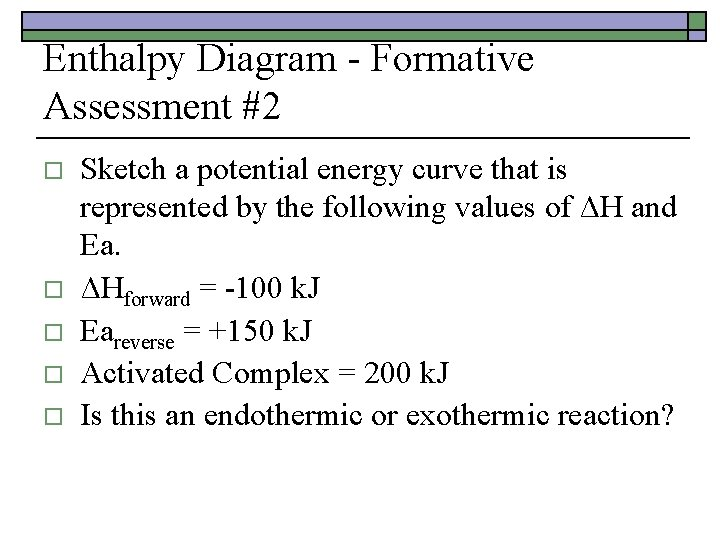Enthalpy Diagram - Formative Assessment #2 o o o Sketch a potential energy curve that is represented by the following values of ΔH and Ea. ΔHforward = -100 k. J Eareverse = +150 k. J Activated Complex = 200 k. J Is this an endothermic or exothermic reaction?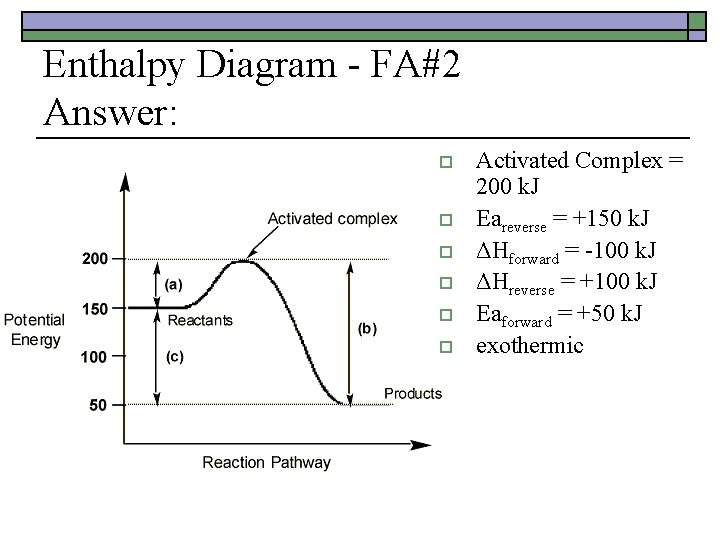Enthalpy Diagram - FA#2 Answer: o o o Activated Complex = 200 k. J Eareverse = +150 k. J ΔHforward = -100 k. J ΔHreverse = +100 k. J Eaforward = +50 k. J exothermic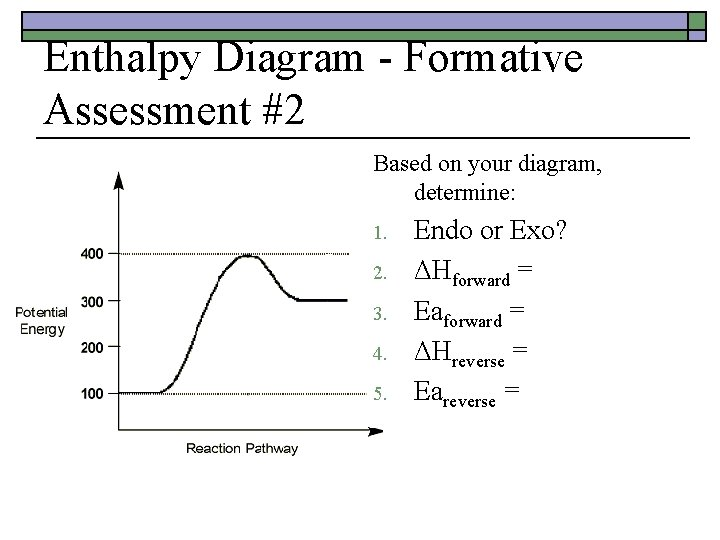Enthalpy Diagram - Formative Assessment #2 Based on your diagram, determine: 1. 2. 3. 4. 5. Endo or Exo? ΔHforward = Eaforward = ΔHreverse = Eareverse =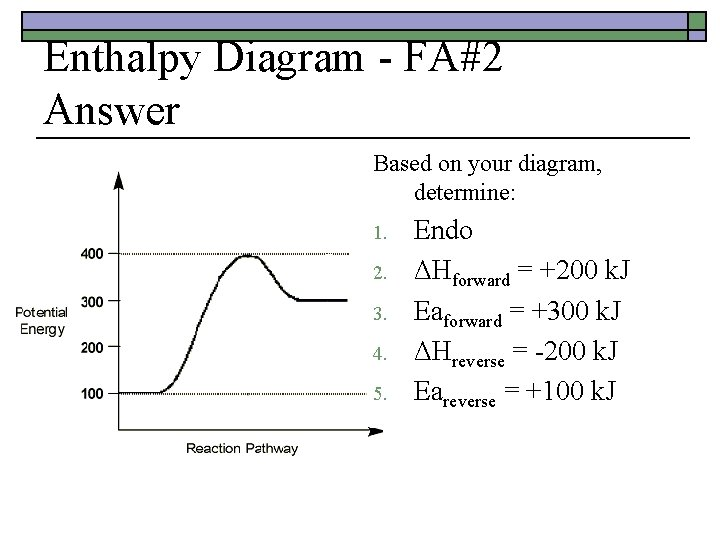Enthalpy Diagram - FA#2 Answer Based on your diagram, determine: 1. 2. 3. 4. 5. Endo ΔHforward = +200 k. J Eaforward = +300 k. J ΔHreverse = -200 k. J Eareverse = +100 k. JCalculating ∆H using Bond Energy 2 H 2 + O 2 2 H 2 O Bonds Formed = exothermic (-) Bonds Broken = endothermic (+) Using Bond Energy Table, determine ∆H. ∆H = -482 k. J (482 k. J released = exothermic)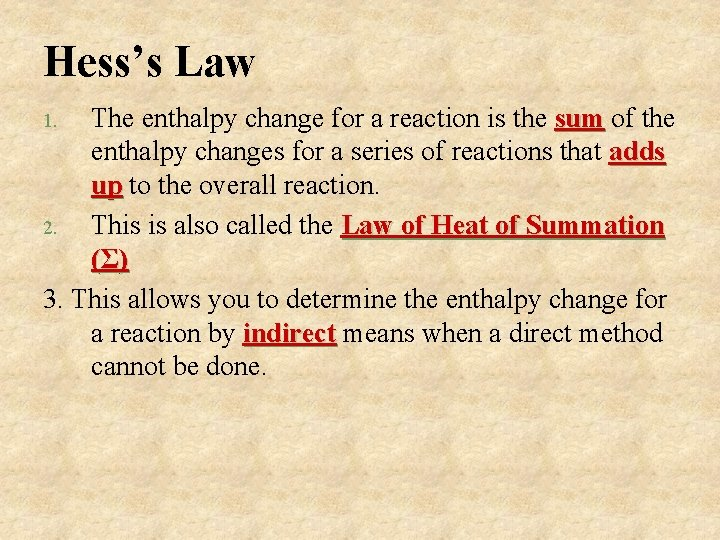Hess’s Law The enthalpy change for a reaction is the sum of the sum enthalpy changes for a series of reactions that adds up to the overall reaction. up 2. This is also called the Law of Heat of Summation (Σ ) 3. This allows you to determine the enthalpy change for a reaction by indirect means when a direct method indirect cannot be done. 1.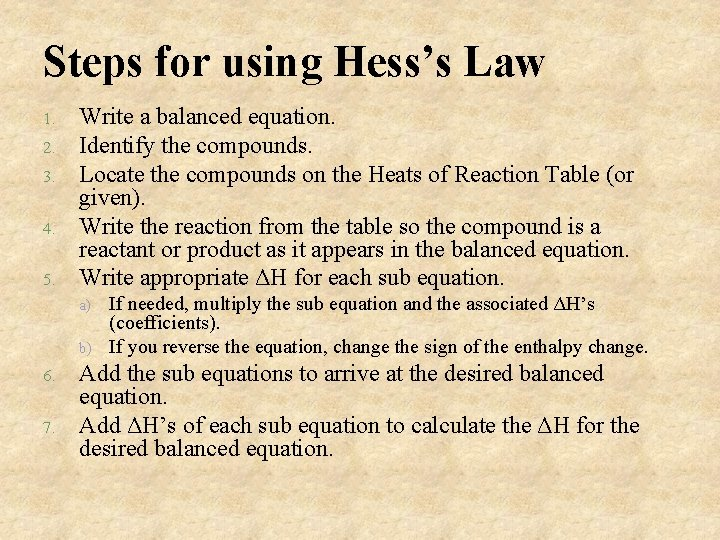Steps for using Hess’s Law 1. 2. 3. 4. 5. Write a balanced equation. Identify the compounds. Locate the compounds on the Heats of Reaction Table (or given). Write the reaction from the table so the compound is a reactant or product as it appears in the balanced equation. Write appropriate ΔH for each sub equation. a) b) 6. 7. If needed, multiply the sub equation and the associated ΔH’s (coefficients). If you reverse the equation, change the sign of the enthalpy change. Add the sub equations to arrive at the desired balanced equation. Add ΔH’s of each sub equation to calculate the ΔH for the desired balanced equation.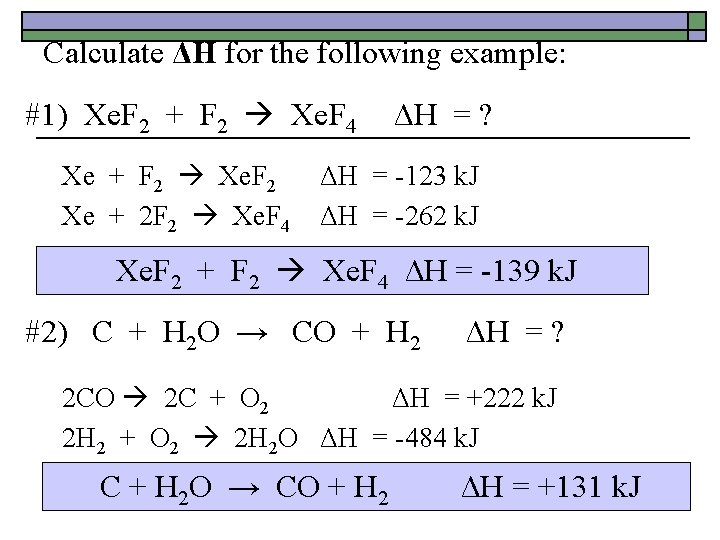Calculate ΔH for the following example: #1) Xe. F 2 + F 2 Xe. F 4 ΔH = ? Xe + F 2 Xe. F 2 ΔH = -123 k. J Xe + 2 F 2 Xe. F 4 ΔH = -262 k. J Xe. F 2 + F 2 Xe. F 4 ΔH = -139 k. J #2) C + H 2 O → CO + H 2 ΔH = ? 2 CO 2 C + O 2 ΔH = +222 k. J 2 H 2 + O 2 2 H 2 O ΔH = -484 k. J C + H 2 O → CO + H 2 ΔH = +131 k. JCalculate ΔH for the following example: #3) CO + O 2 → 2 CO 2 2 C + O 2 2 CO CO 2 C + O 2 ΔH = ? ΔH = -222 k. J ΔH = +394 k. J CO + O 2 → 2 CO 2 ΔH = -394 k. J #4) H 2 O 2 + H 2 → 2 H 2 O ΔH = ? H 2 O + ½ O 2 H 2 O 2 ΔH = +94. 6 k. J 2 H 2 + O 2 2 H 2 O ΔH = -484 k. J H 2 O 2 + H 2 → 2 H 2 O ΔH = -336. 6 k. JCalculate ΔH for the following example: #1) C(s) + H 2 O(g) → CO(g) + H 2(g) H 2 O(g) → H 2(g) + 0. 5 O 2(g) C(s) + 0. 5 O 2 → CO ΔH = +242. 0 k. J ΔH = -111. 0 k. J C(s) + H 2 O(g) → CO(g) + H 2(g) ΔH= +131. 0 k. J Thermochemical Equation: C(s) + H 2 O(g) + 131. 0 k. J → CO(g) + H 2(g)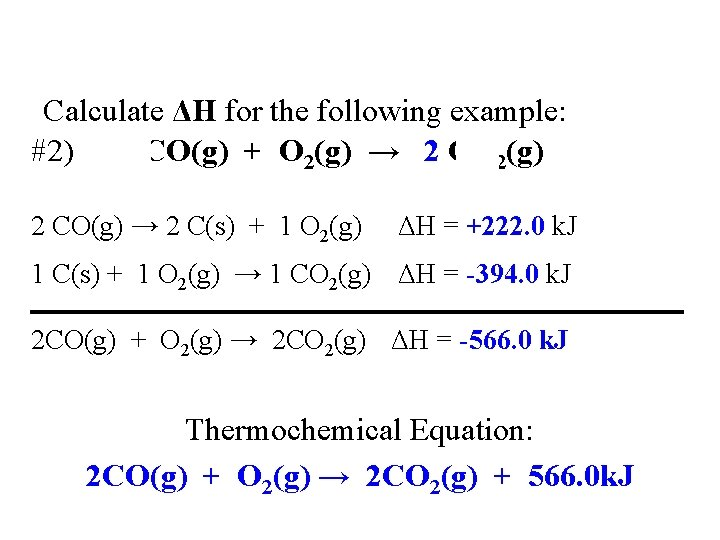Calculate ΔH for the following example: #2) 2 CO(g) + O 2(g) → 2 CO 2(g) 2 CO(g) → 2 C(s) + 1 O ΔH = +222. 0 k. J CO(g) → 0. 5 C 2(g) + C(s) ΔH = +111. 0 k. J 2(g) C(s) + O 2(g) → CO ΔH = -394. 0 k. J 1 C(s) + 1 O 2(g) → 1 CO 2(g) ΔH = -394. 0 k. J 2 CO(g) + O 2(g) → 2 CO 2(g) ΔH = -566. 0 k. J Thermochemical Equation: 2 CO(g) + O 2(g) → 2 CO 2(g) + 566. 0 k. J